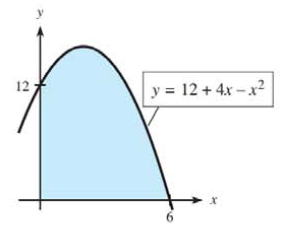Chapter 13, Problem 3T### Mathematical Applications for the ...

11th Edition
Ronald J. Harshbarger + 1 other
ISBN: 9781305108042

#### Solutions

Chapter
Section### Mathematical Applications for the ...

11th Edition
Ronald J. Harshbarger + 1 other
ISBN: 9781305108042
Textbook Problem

# Express the area in Quadrant I under y = 12 + 4 x − x 2 (shaded in the figure) as an integral. Then evaluate the integral to find the area.To determine

To calculate: The area in Quadrant I under the function y=12+4xx2 as an integral for provided figure. Also determine the value of the integral to find the area.

Explanation

Given Information:

The provided function is y=12+4xx2.

The figure provided below shows the graph of the function as,

Formula Used:

The area under the curve y=f(x) over the interval [a,b] is such that,

A=abf(x), where f(x)0

If the function f is continuous on the interval [a,b] then the definite integral of f is,

abf(x)dx=F(b)F(a)

Where, F is any function F(x)=f(x) for all x in the interval [a,b].

The power rule of integral is xndx=xn+1n+1+C, where n1.

Calculation:

Consider the function, y=12+4xx2

From the observation of the graph, the interval in Quadrant I is from x=0 to x=6

### Still sussing out bartleby?

Check out a sample textbook solution.

See a sample solution

#### The Solution to Your Study Problems

Bartleby provides explanations to thousands of textbook problems written by our experts, many with advanced degrees!

Get Started

#### Find the limit: limx2x38x2.

Calculus: An Applied Approach (MindTap Course List)

#### Simplify: (4x3y)(5x+2y)

Elementary Technical Mathematics

#### In Exercises 14, find the slope of the line shown in each figure.

Finite Mathematics for the Managerial, Life, and Social Sciences

#### The appropriate option for the value of (123).

Study Guide for Stewart's Single Variable Calculus: Early Transcendentals, 8th